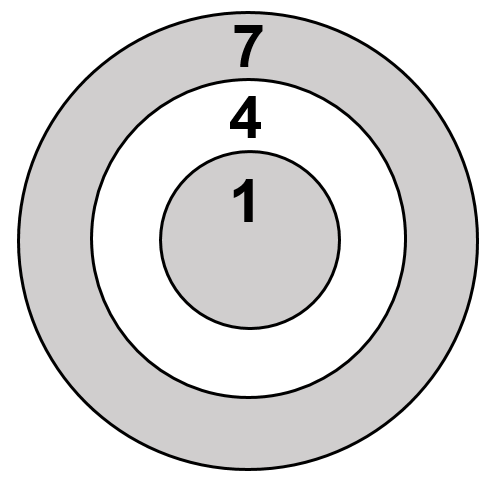Select Page

Each month, a new set of puzzles will be posted.  Come back next month for the solutions and a new set of puzzles, or subscribe to have them sent directly to you.

## ARITH-maze puzzle

In the following ARITH-maze puzzle, go to ‘START’ and proceed through this numeric maze, following instructions as provided, until you get to the ‘FINISH’ box. What number did you finish with?## Feedback

There are more than one way of doing these puzzles and may well be more than one answer.  Please let me and others know what alternatives you find by commenting below.  We also welcome general comments on the subject and any feedback you'd like to give.

If you have a question that needs a response from me or you would like to contact me privately, please use the contact form

## Get more puzzles!

If you've enjoyed doing the puzzles, consider ordering the books;

• Book One - 150+ of the best puzzles
• Book Two - 200+ with new originals and more of your favourites

Both in a handy pocket sized format.   Click here for full details.

## MIND-Xpander maths problems

### PUZZLE A

The second of five consecutive multiples of 11 is removed. The other four are then added together to give 715. What is the lowest multiple of the remaining four? There are two possible solutions.

Solution one

Let x be the unknown multiplier to the multiples.

11x + 0 + 33x + 44x + 55x = 715

143x = 715

x = 5 Checking: 55 + 0 + 165 + 220 + 275 = 715

Solution two

Let x be the lowest multiple multiplier.

11(x) + 0 + 11(x+2) + 11(x+3) + 11(x+4) = 715

11x + 0 + (11x + 22) + (11x + 33) + (11x + 44) = 715

44x + 99 = 715

44x = 616

x = 14

Checking: 154 + 0 + 176 + 187 + 198 = 715

### PUZZLE B

Simplify the following expression: √5 x √15 x √10

Solution## PRIME Numbers puzzle

Throwing 3 darts at the following dartboard and all 3 throws must hit a number on the dartboard, what are your chances that their total will equal a Prime Number? Numbers can be used more than once.1-in-27, There are 3 possible combinations for each of the 3 darts or 27 (3³) different, equally likely combinations.

The only combination that gives you a Prime Number is 1+1+1 = 3. All other combinations are divisible by 3.

## HEXAGON-numeric puzzle (Level 1)